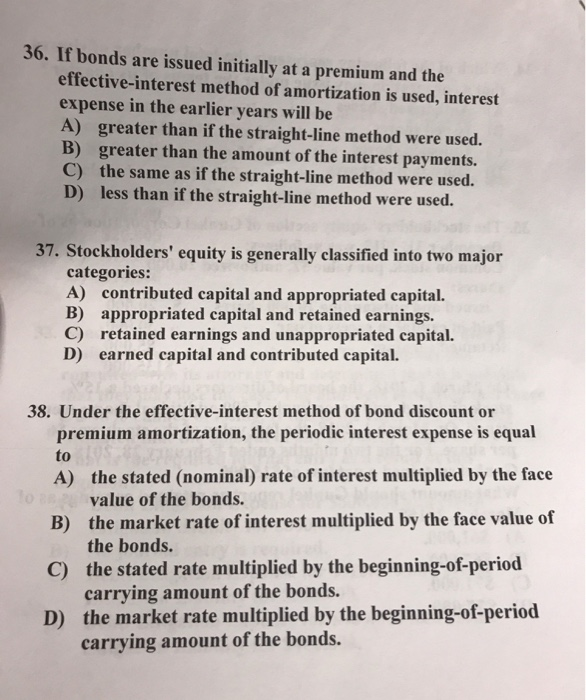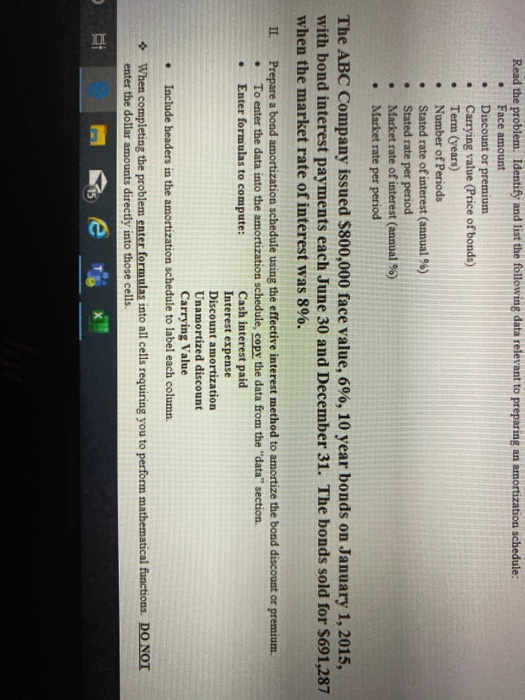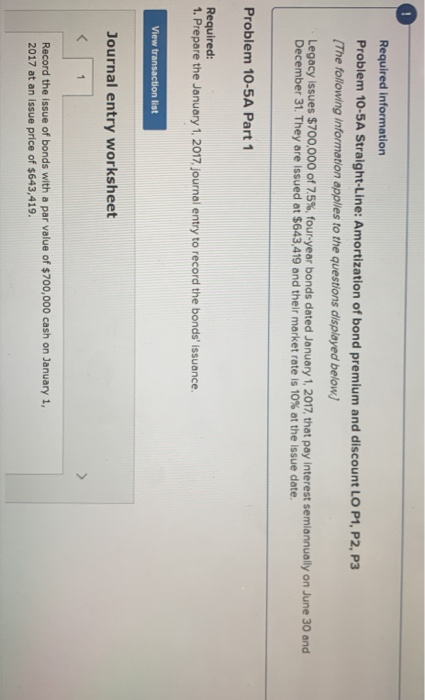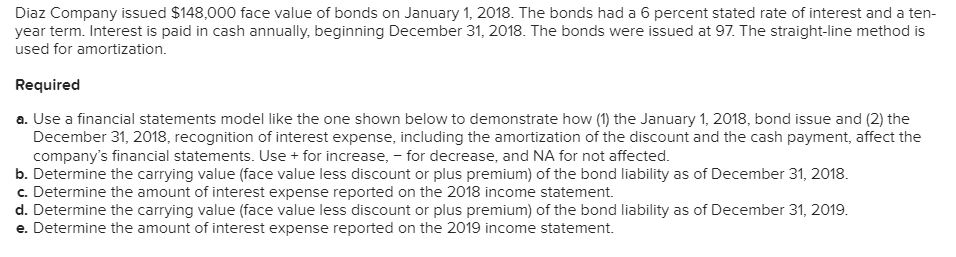Question

Bond Amortization = Bond Discount or Premium / Number of Interest Periods Interest Paid = Face...

• Bond Amortization = Bond Discount or Premium / Number of Interest Periods

• Interest Paid = Face Amount of Bonds x Stated Interest Rate

• Interest Expense = Interest Paid + Discount ( or – Premium) Amortization

On October 1, 2018 ABC issued 5%, 10-year bonds with a face value of \$4,000,000 at 104. Interest is paid on October 1 and April 1, with any premiums or discounts amortized on a straight-line basis. What is interest expense for 2018? Assume ABC Company uses straight-line amortization.

N =

Bond Amortization = Bond Premium / Number of Interest Periods =

Interest Paid = Face Amount of Bonds x Stated Interest Rate =

Interest Expense = Interest Paid - premium amortization =

 N = 20 Bond Premium = (\$4000000*104/100) -40000000 =\$160000 Bond Amortization = Bond Premium / Number of Interest Periods =\$160000/20 = \$8000 Interest Paid = Face Amount of Bonds x Stated Interest Rate = \$4000000*5%/2 =\$100000 Interest Expense = Interest Paid - premium amortization = \$100000-8000 =\$92000

SOLUTION :

Face value of the bond = 4000000

Premium amount = 4000000 * (104 - 100)/100 = 160000

Maturity period of the bond = 10 years.

Coupon rate is 5% semi-annually.

Hence, coupon amount per period of payments

= Interest expense on the bond per period

= 4000000 * 0.05/2

= 100000

Premium of 160000 is to be amortised for 20 semi-annual periods

As per straight line method amortisation per period

= 160000 / 20

= 8000

So, total expense (considered as interest) per period

= Coupon amount - amortised premium

= 100000 -  8000

= 92000.

Bond  issued on October 1, 2018, so in the year 2018 coupon is paid only once on Oct. 1, 2018 itself.

Hence interest expense in 2018 :

= Coupon amount - Amortised premium

= 100000 - 8000

Earn Coins

Coins can be redeemed for fabulous gifts.

Similar Homework Help Questions
• On October 1, 2015, Bartleby Corp. issued 5%, 10-year bonds with a face value of \$3,000,000...

On October 1, 2015, Bartleby Corp. issued 5%, 10-year bonds with a face value of \$3,000,000 at 104%. On October 1 and April 1, interest is paid. Any premiums or discounts are amortized on a straight-line basis. Which of the following will you include in your entry to record the issuance of the bonds? On October 1, 2015, Bartleby Corp. issued 5%, 10-year bonds with a face value of \$3,000,000 at 104%. On October 1 and April 1, interest is...

• 36. If bonds are issued initially at a premium and the effective-interest method of amortization is...36. If bonds are issued initially at a premium and the effective-interest method of amortization is used, interest expense in the earlier years will be A) greater than if the straight-line method were used. B) greater than the amount of the interest payments. C) the same as if the straight-line method were used. D) less than if the straight-line method were used. 37. Stockholders' equity is generally classified into two major categories: A) contributed capital and appropriated capital. B) appropriated...

• Read the problem. Identify and list the following data relevant to preparing an amortization schedule: Face...Read the problem. Identify and list the following data relevant to preparing an amortization schedule: Face amount Discount or premium Carrying value (Price of bonds) Term (years) Number of Periods Stated rate of interest (annual %) Stated rate per period Market rate of interest (annual %) Market rate per period The ABC Company issued \$800,000 face value, 6%, 10 year bonds on January 1, 2015, with bond interest payments each June 30 and December 31. The bonds sold for \$691,287...

• The effective-interest method of bond amortization finds the difference between the ________ times the ________ and...

The effective-interest method of bond amortization finds the difference between the ________ times the ________ and the ________ times the ________. stated interest rate, principal, stated interest rate, carrying value stated interest rate, principal, market interest rate, carrying value stated interest rate, carrying value, market interest rate, principal market interest rate, carrying value, market interest rate, principal The International Financial Reporting Standards require the use of ________. any method of amortization of bond premiums the straight-line method of amortization of...

• Required information Problem 10-5A Straight-Line: Amortization of bond premium and discount LO P1, P2, P3 The...Required information Problem 10-5A Straight-Line: Amortization of bond premium and discount LO P1, P2, P3 The following information applies to the questions displayed below] Legacy issues \$70o,000 of 7.5% four-year bonds dated January 1, 2017, that pay interest semiannually on June 30 and December 31. They are issued at \$643,419 and their mearket rate is 10 % at the issue date Problem 10-5A Part 1 Required: 1. Prepare the January 1, 2017, journal entry to record the bonds' issuance. View...

• Diaz Company issued \$84,000 face value of bonds on January 1, 2018. The bonds had a...

Diaz Company issued \$84,000 face value of bonds on January 1, 2018. The bonds had a 8 percent stated rate of interest and a ten-year term. Interest is paid in cash annually, beginning December 31, 2018. The bonds were issued at 98. The straight-line method is used for amortization. Required Use a financial statements model like the one shown below to demonstrate how (1) the January 1, 2018, bond issue and (2) the December 31, 2018, recognition of interest expense,...

• The Cessna Aircraft Company has outstanding an issue of 4% convertible bonds that mature October 1, 2024. Suppose the bo...

The Cessna Aircraft Company has outstanding an issue of 4% convertible bonds that mature October 1, 2024. Suppose the bonds are dated October 1, 2016, and pay interest each April 1 and October 1. The bond information is as follows: Maturity (face) value - \$100,000 Stated interest rate – 4% Interest paid – Semiannually Market interest rate at the time of issuance – 5% Requirements Assume the bonds are issued at a price of 93.5. Using the straight-line method of...

• Exercise 10-2 Straight-Line: Amortization of bond discount LO P2 Tano issues bonds with a par value...

Exercise 10-2 Straight-Line: Amortization of bond discount LO P2 Tano issues bonds with a par value of \$92,000 on January 1, 2017. The bonds’ annual contract rate is 10%, and interest is paid semiannually on June 30 and December 31. The bonds mature in three years. The annual market rate at the date of issuance is 12%, and the bonds are sold for \$87,480. 1. What is the amount of the discount on these bonds at issuance? 2. How much...

• Please answer in table form only for easy understanding. Thanks. Diaz Company issued \$148,000 face...Please answer in table form only for easy understanding. Thanks. Diaz Company issued \$148,000 face value of bonds on January 1, 2018. The bonds had a 6 percent stated rate of interest and a ten- year term. Interest is paid in cash annually, beginning December 31, 2018. The bonds were issued at 97. The straight-line method is used for amortization Required a. Use a financial statements model like the one shown below to demonstrate how (1) the January 1,...

• Diaz Company issued \$122,000 face value of bonds on January 1, 2018. The bonds had a...

Diaz Company issued \$122,000 face value of bonds on January 1, 2018. The bonds had a 5 percent stated rate of interest and a ten-year term. Interest is paid in cash annually, beginning December 31, 2018. The bonds were issued at 99. The straight-line method is used for authorization. Determine the carrying value (face value less discount or plus premium) of the bond liability as of December 31, 2018. Determine the amount of interest expense reported on the 2018 income...